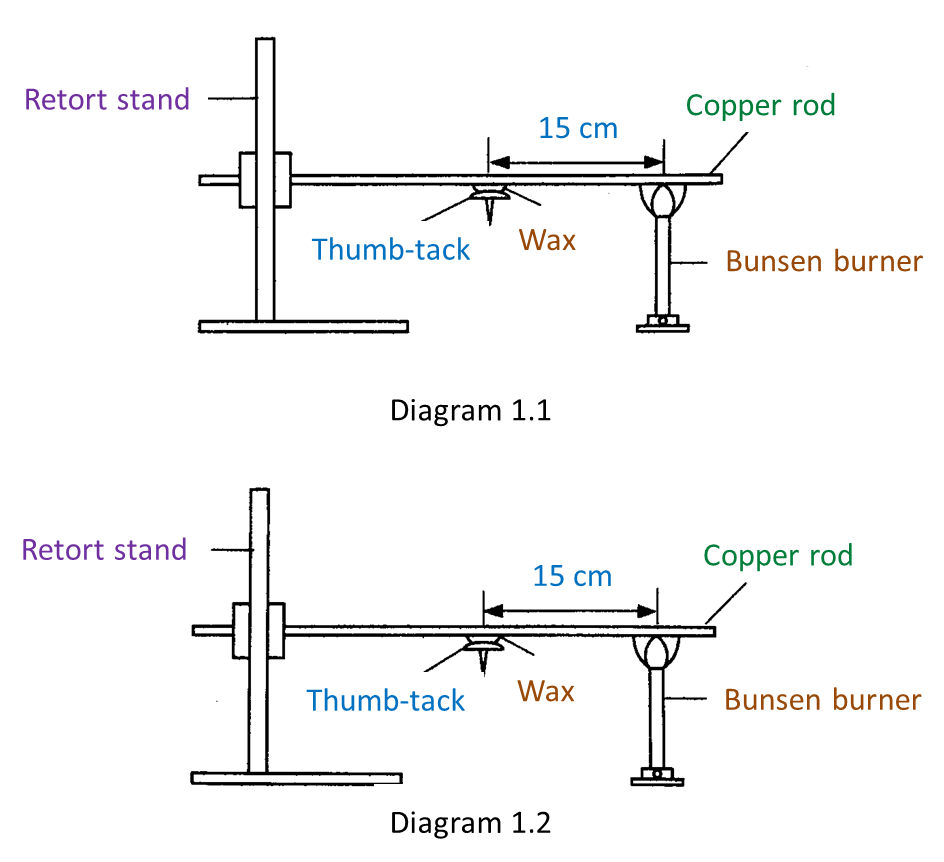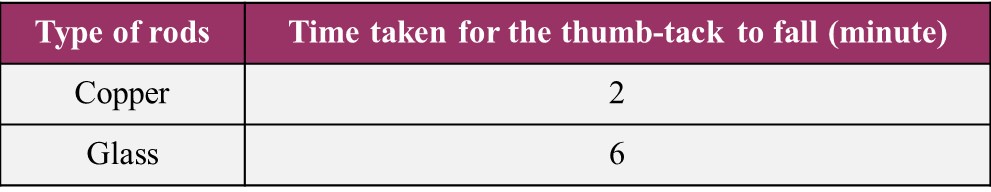# 4.9.4 Properties and Uses of Metals and Non-metals (Structured Questions)

Question 1:
Diagram 1.1 and Diagram 1.2 show an experiment to study the heat conductivity between metal and non-metal.Table shows the time taken for the thumb-tack to fall after both rods are heated.(a) State one hypothesis for this experiment. [1 mark]

(b) State the variables in this experiment.
(i) Constant variable [1 mark]
(ii) Manipulated variable [1 mark]

(c) Based on the Table, which rod is good heat conductor? [1 mark]

(d) Glass is a non-metal substances. Based on this experiment, state the operational definition for non-metal. [1 mark]